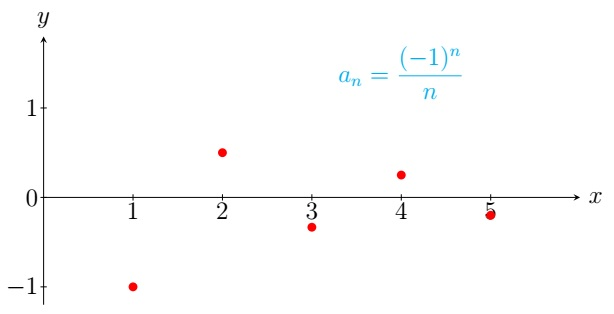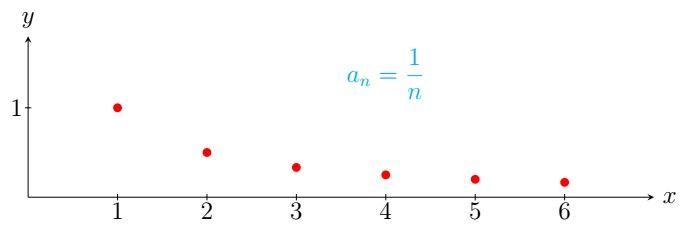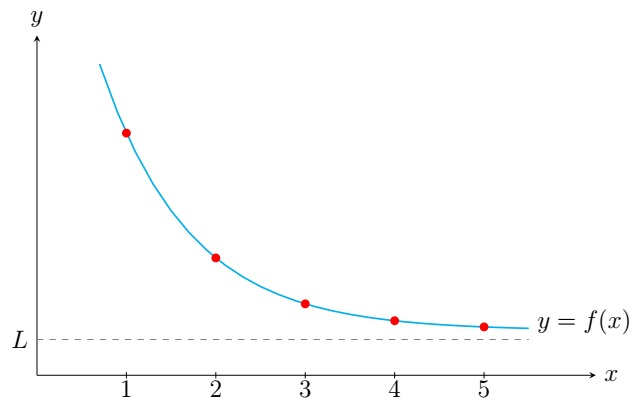Calculus II Home

## Sequences

A sequence $$\{a_n\}$$ of real numbers is a function $$f:\mathbb N\to \mathbb R$$ such that $$f(n)=a_n$$. Sometimes it is denoted by $$\{a_n\}_{n=1}^{\infty}$$ or by a few terms with a certain pattern $$\{a_1,a_2,a_3,\ldots\}$$. A sequence can also be defined recursively.Example.

1. First four terms of the sequence $$\left\lbrace \frac{(-1)^n}{n}\right\rbrace$$ are $$-1,\frac{1}{2},-\frac{1}{3},\frac{1}{4}$$.

2. $$\{1,\frac{1}{2},\frac{1}{3},\frac{1}{4},\ldots\}$$ can be written as $$\left\lbrace \frac{1}{n}\right\rbrace$$.

3. The Fibonacci sequence $$\{F_n\}_{n=0}^{\infty}$$ is defined by $F_n=F_{n-1}+F_{n-2},\; n\geq 2,\; F_0=0,\; F_1=1.$

Definition. A sequence $$\{a_n\}$$ converges to a real number $$L$$ if $$x_n$$ can be made arbitrarily close to $$L$$ by taking sufficiently large $$n$$ (for details, see the $$\varepsilon-N$$ definition). It is denoted by $$\displaystyle\lim_{n\to \infty} a_n=L$$ or $$a_n\to L$$ as $$n\to \infty$$. The sequence $$\{a_n\}$$ diverges if it is not convergent.Example.

1. The sequence $$\left\lbrace \frac{1}{n}\right\rbrace$$ converges to $$0$$.

2. $$\{ n^2 \}$$ diverges to $$\infty$$.

3. $$\{(-1)^n\}$$ diverges as its terms oscillate between $$1$$ and $$-1$$.

Note that if a sequence $$\{x_n\}$$ diverges, its terms oscillate indefinitely like that of $$\{(-1)^n\}$$ or it diverges to $$\pm \infty$$.

Theorem. If $$a_n=f(n)$$ for integers $$n$$ and $$\displaystyle\lim_{x\to \infty} f(x)=L$$, then $$\displaystyle\lim_{n\to \infty} a_n=L$$.Example. Show that $$\displaystyle\lim_{n\to \infty} \frac{n+\tan^{-1}n}{n}=1$$.

Solution. Let $$f(x)=\displaystyle\frac{x+\tan^{-1}x}{x}$$. Note that $$f(n)$$ is the $$n$$th term of the sequence $$\left\lbrace \displaystyle\frac{n+\tan^{-1}n}{n} \right\rbrace$$. $\displaystyle\lim_{x\to \infty} f(x)= \displaystyle\lim_{x\to \infty} \frac{x+\tan^{-1}x}{x} = \displaystyle\lim_{x\to \infty} \frac{1+\frac{1}{1+x^2}}{1} =1 \;\;\left(\text{by l'Hospitals's Rule} \right).$ Thus $$\displaystyle\lim_{n\to \infty} \frac{n+\tan^{-1}n}{n}=1$$, i.e., the sequence $$\left\lbrace \displaystyle \frac{n+\tan^{-1}n}{n} \right\rbrace$$ converges to $$1$$.

Algebra of limits for sequences:
Theorem. The following are true for any convergent sequences $$\{a_n\}$$ and $$\{b_n\}$$:

1. $$\displaystyle\lim_{n\to \infty}(a_n\pm b_n)=\left(\displaystyle\lim_{n\to \infty} a_n \right)\pm \left(\displaystyle\lim_{n\to \infty} b_n \right)$$.

2. $$\displaystyle\lim_{n\to \infty}(ca_n)=c\displaystyle\lim_{n\to \infty} a_n$$ for all real numbers $$c$$.

3. $$\displaystyle\lim_{n\to \infty} a_nb_n=\left(\displaystyle\lim_{n\to \infty} a_n \right) \left(\displaystyle\lim_{n\to \infty} b_n \right)$$.

4. $$\displaystyle\lim_{n\to \infty} \frac{a_n}{b_n}=\frac{\displaystyle\lim_{n\to \infty} a_n}{\displaystyle\lim_{n\to \infty} b_n}$$ whenever $$\displaystyle\lim_{n\to \infty} b_n\neq 0$$.

5. $$\displaystyle\lim_{n\to \infty} a_n^p=\left(\displaystyle\lim_{n\to \infty} a_n \right)^p$$ for all real numbers $$p>0$$ whenever $$a_n>0$$.

Note that the convergence in the assumption is crucial. For $$\{a_n\}=\{\frac{1}{n}\}$$ and $$\{b_n\}=\{n\}$$, we have $$\{a_nb_n\}=\{1\}$$. But $\displaystyle\lim_{n\to \infty} a_nb_n=1\neq 0\cdot \infty\ =\left(\displaystyle\lim_{n\to \infty} a_n \right) \left(\displaystyle\lim_{n\to \infty} b_n\right).$

Example. Determine if the sequence $$\{a_n\}$$ converges or diverges.
(a) $$a_n=\displaystyle\frac{n^3}{n^3+1}$$, (b) $$a_n=\displaystyle\frac{n^3}{n+1}$$, (c) $$a_n=\displaystyle\sqrt{\frac{n+1}{9n+1}}$$.

Solution. (a) $$\displaystyle\lim_{n\to \infty} a_n=\displaystyle\lim_{n\to \infty}\frac{n^3}{n^3+1} =\displaystyle\lim_{n\to \infty}\frac{1}{1+\frac{1}{n^3}} =\displaystyle\frac{1}{1+\displaystyle\lim_{n\to \infty}\frac{1}{n^3}} =\displaystyle\frac{1}{1+0} =1.$$ Thus $$\left\lbrace \displaystyle\frac{n^3}{n^3+1} \right\rbrace$$ converges to $$1$$.

(b) $$\displaystyle\lim_{n\to \infty} a_n=\displaystyle\lim_{n\to \infty}\frac{n^3}{n+1} =\displaystyle\lim_{n\to \infty}\frac{n^2}{1+\frac{1}{n}} =\infty.$$ Thus $$\left\lbrace \displaystyle\frac{n^3}{n^3+1} \right\rbrace$$ diverges to $$\infty$$.

(c) $$\displaystyle\lim_{n\to \infty} a_n =\displaystyle\lim_{n\to \infty}\sqrt{\frac{n+1}{9n+1}} =\displaystyle\sqrt{ \displaystyle\lim_{n\to \infty}\frac{n+1}{9n+1}} =\displaystyle\sqrt{ \displaystyle\lim_{n\to \infty}\frac{1+\frac{1}{n}}{9+\frac{1}{n}}} =\displaystyle\sqrt{ \frac{1+0}{9+0}} =\frac{1}{3}.$$ Thus $$\left\lbrace \displaystyle\sqrt{\frac{n+1}{9n+1}} \right\rbrace$$ converges to $$\frac{1}{3}$$.

Theorem. If $$\{a_n\}$$ is convergent and $$f$$ is a continuous function, then $\displaystyle\lim_{n\to \infty} f(a_n)=f\left( \displaystyle\lim_{n\to \infty} a_n \right).$

Example. Since $$f(x)=\cos x$$ is a continuous function, $\displaystyle\lim_{n\to \infty} \cos\left( \frac{2}{n} \right)=\cos\left( \displaystyle\lim_{n\to \infty} \frac{2}{n} \right)=\cos 0=1.$ Thus $$\left\lbrace \cos\left( \frac{2}{n} \right) \right\rbrace$$ converges to $$1$$.

Theorem.(Sandwich Theorem) Suppose $$a_n\leq b_n\leq c_n$$ for all natural numbers $$n$$. If both $$\{a_n\}$$ and $$\{c_n\}$$ converge to $$L$$, then $$\{b_n\}$$ also converges to $$L$$.

Example. Show that $$\displaystyle\lim_{n\to \infty} \frac{\sin(3n)}{n}=0$$.

Solution. First note that $$-1\leq \sin(3n) \leq 1$$ for all real numbers $$n$$. Then for all natural numbers $$n$$, $-\frac{1}{n}\leq \frac{\sin(3n)}{n} \leq \frac{1}{n}.$ Since $$\displaystyle\lim_{n\to \infty} \frac{-1}{n}=\displaystyle\lim_{n\to \infty} \frac{1}{n}=0$$, we have $$\displaystyle\lim_{n\to \infty} \frac{\sin(3n)}{n}=0$$ by the Sandwich Theorem.

As consequences of the Sandwich Theorem, we have the following results:

1. If $$\displaystyle\lim_{n\to \infty} |a_n|=0$$, then $$\displaystyle\lim_{n\to \infty} a_n=0$$.

2. $$\left\lbrace r^n \right\rbrace$$ is convergent if $$-1< r\leq 1$$ and divergent otherwise. In particular, $\lim_{n\to \infty} r^n= \begin{cases} 0 & \text{if } -1< r <1 \\ 1 & \text{if } r=1. \end{cases}$

(a) $$-|a_n|\leq a_n \leq |a_n|$$ for all natural numbers $$n$$. Now apply the Sandwich Theorem.

(b) If $$r=1$$, then $$\left\lbrace r^n \right\rbrace=\{1\}$$ is convergent. Suppose $$-1< r< 1$$. Then $$0\leq |r|< 1$$. Since $$\displaystyle\lim_{x\to \infty} |r|^x=0$$, $$\displaystyle\lim_{n\to \infty} |r|^n=0$$. Since $$|r|^n=|r^n|$$, $$\displaystyle\lim_{n\to \infty} r^n=0$$ by (a).
For $$r>1$$, $$\displaystyle\lim_{x\to \infty} r^x=\infty$$ and hence $$\displaystyle\lim_{n\to \infty} r^n=\infty$$. For $$r=-1$$, note that $$\{(-1)^n\}$$ is divergent as its terms oscillate between $$1$$ and $$-1$$. Similar arguments can be applied for $$r<-1$$.

Example. (a) Since $$\displaystyle\lim_{n\to \infty} \left| \frac{(-1)^n}{n} \right|=\displaystyle\lim_{n\to \infty} \frac{1}{n}=0$$, $$\displaystyle\lim_{n\to \infty} \frac{(-1)^n}{n}=0$$.

(b) $$\displaystyle\lim_{n\to \infty} \frac{2^n(-1)^n}{3^n}=\displaystyle\lim_{n\to \infty} \left( -\frac{2}{3} \right)^n=0$$ as $$-1<-\frac{2}{3}\leq 1$$. $$\displaystyle\lim_{n\to \infty} \left(\frac{3}{2} \right)^n$$ does not exist as $$\frac{3}{2}>1$$.

Definition. A sequence $$\{a_n\}$$ is bounded if there exists a real number $$M$$ such that $$|a_n|\leq M$$ for all natural numbers $$n$$.

Example. $$\{\frac{1}{n}\}$$ is bounded since $$|\frac{1}{n}|\leq 1$$ for all natural numbers $$n$$.

Theorem. A convergent sequence is bounded.

Definition. A sequence $$\{a_n\}$$ is increasing if $$a_{n+1} > a_n$$ for all natural numbers $$n$$ and decreasing if $$a_{n+1}< a_n$$ for all natural numbers $$n$$.

Theorem.(Monotone Convergence Theorem)

1. An increasing sequence that is bounded above is convergent.

2. A decreasing sequence that is bounded below is convergent.

Note that the Monotone Convergence Theorem is also true for non-decreasing and non-increasing sequences respectively.

Example.

1. $$\{\frac{1}{n}\}$$ is a decreasing sequence as $$a_{n+1}=\frac{1}{n+1}<\frac{1}{n}=a_n$$ for all natural numbers $$n$$. Also $$\{\frac{1}{n}\}$$ is bounded below by $$0$$ as $$0<\frac{1}{n}=a_n$$ for all natural numbers $$n$$. Since $$\{\frac{1}{n}\}$$ is decreasing and bounded below, it is convergent by the Monotone Convergence Theorem.

2. $$\{x_n\}$$ where $$x_1=\sqrt{2}$$ and $$x_n=\sqrt{2+x_{n-1}},\;n\geq 2$$.
We show $$\{x_n\}$$ is increasing by induction. First note that $x_1=\sqrt{2}< \sqrt{2+\sqrt{2}}=\sqrt{2+x_1}=x_2.$ Assume $$x_{n-1}< x_n$$ for some $$n\in \mathbb N$$. We show $$x_{n} < x_{n+1}$$. Note that \begin{align*} & x_{n+1}^2-x_n^2=(2+x_n)-(2+x_{n-1})=x_n-x_{n-1}\\ \implies & x_{n+1}-x_n=(x_n-x_{n-1})/(x_{n+1}+x_n) \end{align*} Since $$x_{n-1} < x_n$$ and $$x_n,x_{n+1} > 0$$, we have $$x_{n+1}-x_n=(x_n-x_{n-1})/(x_{n+1}+x_n) > 0$$, i.e., $$x_{n} < x_{n+1}$$.
We show $$\{x_n\}$$ is bounded above also by induction. Note that $$x_1=\sqrt{2}<3$$ and $$x_2=\sqrt{2+\sqrt{2}}<3$$. Assume $$x_n < 3$$ for some $$n\in \mathbb N$$. We show $$x_{n+1} < 3$$. Note that $x_{n+1}=\sqrt{2+x_n}<\sqrt{2+3} < 3.$ By mathematical induction $$x_n < 3$$ for all natural numbers $$n$$.
Since $$\{x_n\}$$ is an increasing sequence bounded above by $$3$$, it is convergent by the MCT. Suppose $$\displaystyle\lim_{n\to \infty} x_n=L$$. To find $$L$$, note that $$x_n^2=2+x_{n-1},\;n\geq 2.$$ Taking limit of both sides we get $\displaystyle\lim_{n\to \infty} x_n^2=\displaystyle\lim_{n\to \infty} (2+x_{n-1}) \implies (\displaystyle\lim_{n\to \infty} x_n)^2=2+ \displaystyle\lim_{n\to \infty} x_{n-1} \implies L^2=2+L \implies L=-1,2.$ Since $$x_n>0$$ for all natural numbers $$n$$, $$L\geq 0$$. Thus $$L=2$$, i.e., $$\displaystyle\lim_{n\to \infty} x_n=2$$.

Last edited